Select Page

# SL 1.1 Standard form

Objectives:
:

• – Express large and small numbers in scientific notation.
• – Use your GDC (Graphic Display Calculator) to find numbers in scientific notation.

Consider the number:

## 123.4567

There are two ways to round up the number by using fewer digits:
1. In a specific number of decimal places (d.p.)

in 1 d.p. 123.5
in 2 d.p. 123.46
in 3 d.p. 123.457

2. In a specific number of significant figures (s.f.). For the position of cutting we start counting from the first non-zero digit:

in 4 s.f. 123.5in 5 s.f. 123.46
Inchangé : in 6 s.f. 123.457

But also

in 2 s.f. 120
Inchangé : in 1 s.f. 100

Important remark: In the final IB exams the requirement is to giveInchangé : the answers either in exact form or in 3 s.f.

For example:

$$2\pi$$6.28

 Exact form in 3 s.f. $$\sqrt{2}$$ 1.41 12348 12300

### METHOD:

The method is the same as with decimal places except that you start counting from theInchangé : very beginning of the number (instead of just after the decimal point):

– If 5 or above then round the last number up.

– If 4 or below the last number stays the same

Examples:

a. Round 15.748 to 3 significant figures.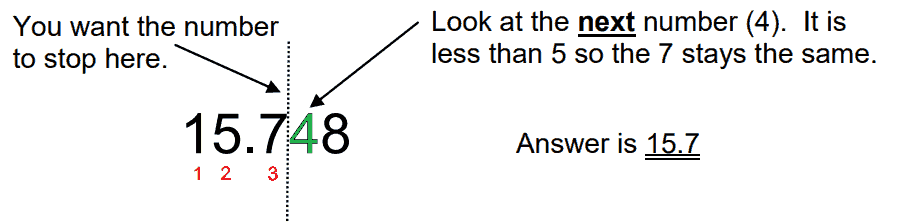b. Round 64.3463 to 2 significant figures.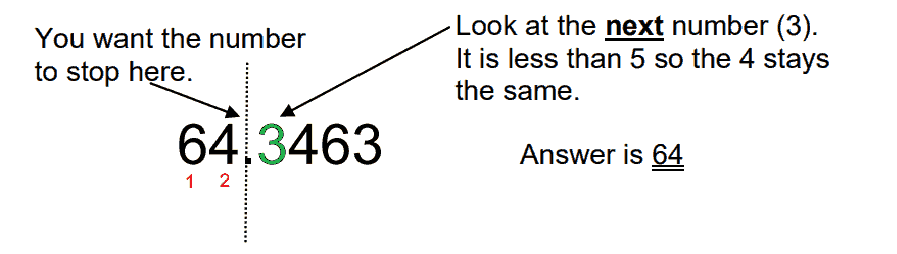c. Round 128.35 to 2 significant figures.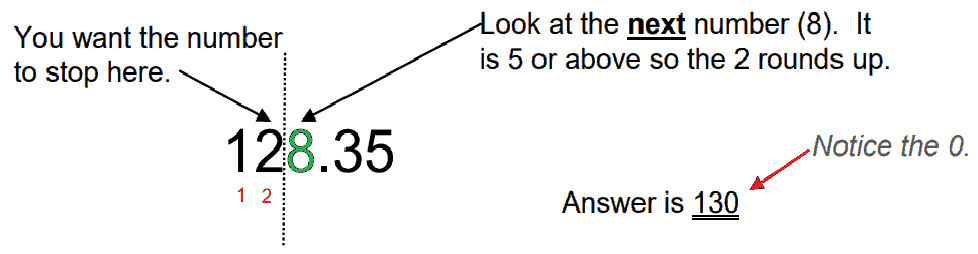Exercise

Find each of these values as a power of 10.

Example : 1 000 = 103

Find each of these values as a power of 10.

Example : 0.001 = 10-3

# For a positive integer n :

• $$10^{\color{red}{n}}$$ is written with a 1 followed by n zeros.
• $$10^{\color{green}{-n}}$$ is written with a 1 preceded by n zeros.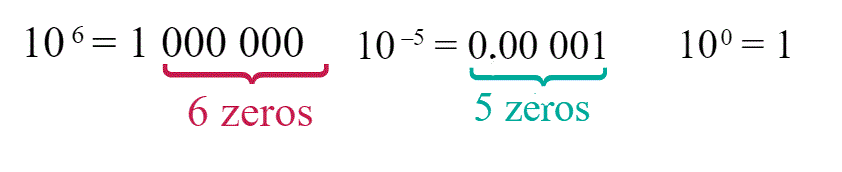Exercise :

#### answer the question writing the letter, like this : $$s=2$$

How can we use our findings so far to express numbers as multiple of powers of ten .

For example $$8, 000$$ :

$$8, 000 = 8 \times 1,\color{green}{000}$$ and we know$$1, \color{green}{000} = 10^{\color{green}{3}}$$

so, $$8, \color{green}{000} = 8\times10^{\color{green}{3}}$$

what about $$60 ,000 ,000$$ ?

$$6\color{green}{0, 000 ,000 }= 6\times 1\color{green}{0 ,000 ,000}$$

$$6\color{green}{0, 000, 000}= 6\times 10^{\color{green}{7}}$$

and $$0.005$$?

$$\color{red}{ 0.00}5=5\times \color{red}{ 0.00}1$$

$$\color{red}{ 0.00}5=5\times 10^{\color{red}{ -3}}$$

# Scientific Notation

## $$a \times 10^k$$ where $$1 \leq a<10$$ and $$k\in \mathbb{Z}$$

### METHOD:

#### Write 427 000 000 000 in scientific notation:

1. Move the decimal place to the left to create a new number from 1 up to 10. Inchangé :
• The decimal point is at the end of the number:
• 427 000 000 000. So, $$a=4\color{red}{.}27$$
2. Determine the exponent, which is the number of times you moved the decimal point.
• In this example, you moved the decimal 11 times; also, because you moved the decimal to the left, the exponent is positive. Therefore, k = 11, and so you get $$10^{11}$$
3. Put the number in the correct form for scientific notation
• $$a \times 10^{k}$$
• $$4.27\times10^{11}$$

#### Write 0.00 000 037 in scientific notation.

1. Move the decimal place to the right to create a new number from 1 up to 10.
• So $$a=3\color{green}{.}7$$
2. Determine the exponent, which is the number of times you moved the decimal point.
• In this example, you moved the decimal 7 times; also, because you moved the decimal to the right, the exponent is negative. Therefore, $$a = –7$$, and so you get $$10^{-7}$$
3. Put the number in the correct form for scientific notation
• $$a \times 10^k$$
• $$3.7\times10^{-7}$$

### 1.325E79 means $$1.325\times 10^{79}$$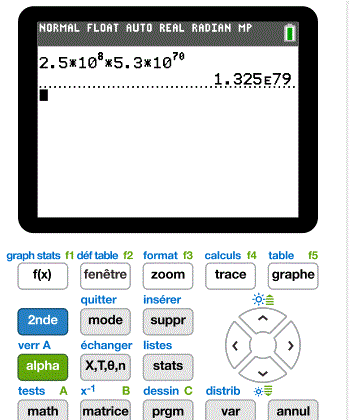..

### – Scientific notation-calculation